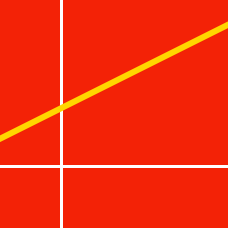Geometry

# Equation of a Line: Level 1 Challenges

Consider the line given by the equation$y=\frac{5}{2}x+30.$ Let $$a$$ and $$b$$ be the $$x$$-intercept and $$y$$-intercept of this line. What is the value of $$a+b$$?

Two lines $$y-ax=2$$ and $$x+23y=138$$ are perpendicular to each other. What is the value of $$a$$?

Find the equation of the line which has a slope of $$-\frac{3}{4}$$ and forms a triangle with the positive coordinate axes such that the triangle has an area of 24 square units.

If three points $$A=(k,k+2),$$ $$B=(0,k-6)$$ and $$C=(k-4,k)$$ all lie on the same line, what is the value of $$k?$$

The graph of the line $$y=ax+b$$ passes through the point $$(2, 90)$$. Additionally, traveling along the line, if $$x$$ decreases by $$2$$, then $$y$$ decreases by $$4$$. What is the sum of the $$x$$-intercept and the $$y$$-intercept?

×

Problem Loading...

Note Loading...

Set Loading...